Posted by: pixelero | December 15, 2014

## Linear and Radial Blur with HTML5

Hi again,

This new post is an html5 implementation of my older
flash demo of applying a linear blur
,
e.g. to blur an image in such a way that some areas in it remain sharp.
The solution in the new one is however slightly different: instead of sampling nearby pixels, I have used context.setTransform() with a Matrix defined so that the same effect is achieved. The old flash demo had only a linear mode, here’s also added a radial or circular version.
The aligning of three source points (p0,p1 and p2) with three destination points (q0,q1 and q2) is calculated by solving a matrix equation. Each point form a row in the matrices. Parameters a,b,c,d,e and f refer to parameters of context.setTransform():$\begin{bmatrix} a & b & 1 \\ c & d & 1 \\ e & f & 1 \\ \end{bmatrix}=\begin{bmatrix} p_{0,x} & p_{0,y} & 1 \\ p_{1,x} & p_{1,y} & 1 \\ p_{2,x} & p_{2,y} & 1 \\ \end{bmatrix}^{-1} \times \begin{bmatrix} q_{0,x} & q_{0,y} & 1 \\ q_{1,x} & q_{1,y} & 1 \\ q_{2,x} & q_{2,y} & 1 \\ \end{bmatrix}$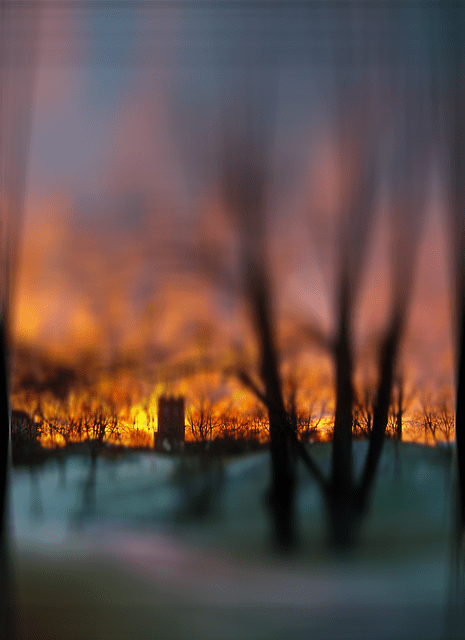View the Source Code.

Posted by: pixelero | November 26, 2014

## Spiral Moiré

It started by creating a Low-discrepancy, or quasi-random sequence filling a circular area along a spiral.
Using a polar formula$x=\sqrt{\theta} \cdot cos(f \cdot \sqrt{\theta}), y=\sqrt{\theta} \cdot sin(f \cdot \sqrt{\theta})$
gives a nice even-looking result: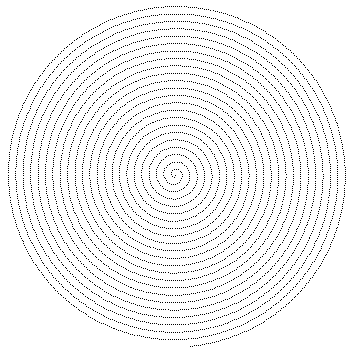When both the number of pixels and the rotations around the origin are increased, things start to get artistic. These are the results of choosing number of pixels to 75000, and value of ‘factor’ was 11.1:Demo

Posted by: pixelero | November 16, 2014

## Median Filter for html5/js

Median Filter – my implementation for html5/canvas and javascript.

The naive approach is to collect all the pixels from the surrounding area, sort them, and return the middle, median, value. Especially with web-based techniques this becomes computationally a very heavy operation, so I’ve used a couple of optimizations to boost up the performance.

First is that, when analyzing the image, only small changes to the sample are made. For the update e.g. taking one pixel step, it’s enough to remove the pixels from the left edge and add the new ones at right edge. This reduces the number of calculations in a general case from NxN to 2N: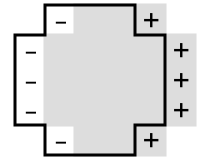The second optimization comes to the way of sorting the pixel data. Since the color data has discrete, integer values from a limited 0…255 range, I’ve chosen a histogram to do the sorting with, compare this to like for instance counting sort. And because the changes in data are small, I keep track of the position of the median.The median’s position is updated on how data changes. There’s a couple of simple rules to follow:
If the added value is smaller than current median, the median should take a step downwards or to the previous bin.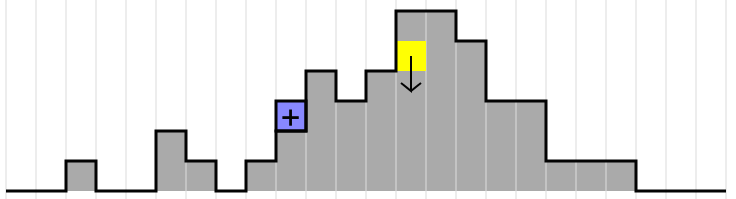Likewise if a removed value is smaller, the median should take a step upwards.
If the added or removed value is larger, it doesn’t affect the median position at all. Remember that at each step there are as many removed as added values.

In a general case the changes in median’s position remained small, even plus-minus zero in most cases. To map a rgb-coordinate from the 3D-color space to a histogram range of course has some disadvantages: several distinctly different colors might ‘hit’ the same histogram bin. To avoid these data collisions and also to have an even distribution in the histogram bins, I’ve first equalized the rgb-channels. And remember this is a greedy algorithm adaption, in cases when median is read from a bin with several entries, the average of these colors is returned.

Of course, if we can sort an array and pick a median, why not try picking up as well the k:th item in order. In the demo you can also set the value to pick to k:th element. If that is set to zero, the result is as picking the minimum, and with 1.0 like the maximum.

Javascript code.

Posted by: pixelero | November 13, 2014

## “How do I generate weighted random numbers?”

Hi there folks!

It looks like one link leading most visitors to this blog is a discussion on a programming forum and that this old post of mine about random numbers is among the most popular ones. No wonder, there’s not much information about how to modify the distribution of random variables easily available.
In this case the basic question is how to pick random variables on a certain range 0…N so that the main weight is one a desired value A in that range e.g. Meaning that if A is near zero, the expected value is small, and vice versa large when A approaches N.

Solution 1:
First thing that comes to mind is that taking the maximum of two Math.randoms returns a result near 1.0, and likewise the minimum remains near zero.Now if we take an linear average of these two with respect to the desired center value A we get a formula:
A=0.3;(1-A)*Math.min(Math.random(),Math.random()) + A*Math.max(Math.random(),Math.random())
Here’s the result of that with A=0.3 on my old demo: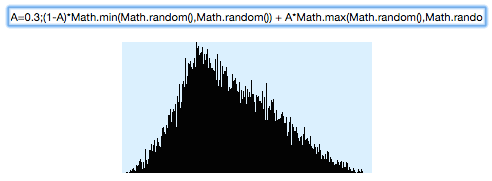Ok, looks like that pretty close to what was asked, although four Math.randoms are needed for one single result. What would this look like a mathematical formula? Well, I’m not going to calculate it but according to probabilistic theories the sum of two distributions is their convolution.
Just try it with different values for A in range 0…1.
Another solution with a more Gaussian form would be like for instance A+(Math.random()+Math.random()-Math.random()-Math.random())/4 although you have to remove the values outside of the range 0 to 1, see also Irwin-Hall distribution.

Solution 2:
Then again, could we possible find a more exact solution for this problem? Let’s now have a look on an analytic solution, how to adapt a random variable to a chosen distribution by a mathematical formula.
First we need a function y=f(x) for the wished probability density. In this case it’s a piecewise defined function:The factor of 2 comes from the fact that the sum of all probabilities, when integrated over 0<x<1 must be equal to 1.0. The integral function of this, aka Cumulative distribution function F(x) or CDF tells us the probability of a random variable is below x: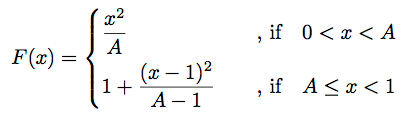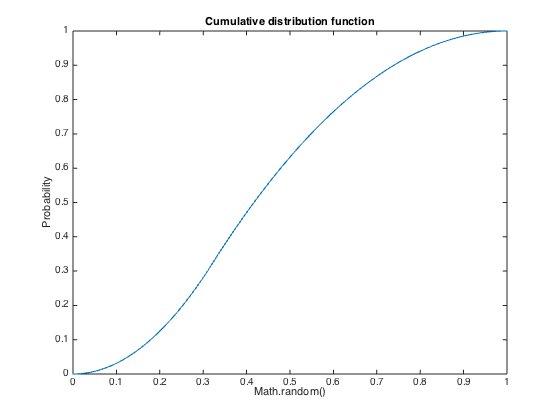The function we were actually looking for, is the inverse of the F(x). In the process of finding out this result, there are two phases which might prove hard to solve, first the integration and then solving the inverse, but now we turned lucky. This is function we’ll apply to a random number to get the distribution we were after: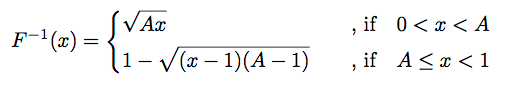Now looking at that formula, who would have guessed this is the result?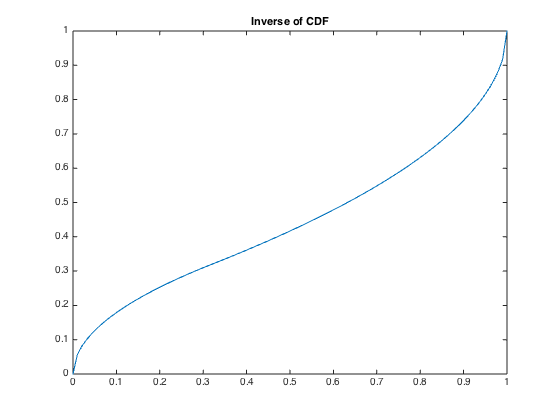Now writing that as a piece of javascript code would be:
A=0.3;x=Math.random(); x<A ? Math.sqrt(A*x) : 1-Math.sqrt((A-1)*(x-1));Comparing it to the first result shows that this has a smoother more obviously linear distribution around value A.

Solution 3:
In both these solutions the probability is at highest at x=A, but the mean of the distribution might not be E(x)=∫x p(x) dx=A.
Let’s choose some distribution with E(x)=A as a starting point, one such would be
A=0.3; x=Math.random(); x<0.5 ? 2*A*x : 2*(x-1)*(1-A)+1That works so that values below x=0.5 are mapped to range 0.0…A and likewise values x>0.5 to range A…1.0: half the probability is now below A and the other half above A. But the distribution on both sides is uniform, and we want the highest probability to X=A?
Now averaging two similar samples doesn’t change the mean value, but puts the highest probability to x=A, and the result is:
A=0.3; x=Math.random()+Math.random(); x<1.0 ? A*x : (x-2)*(1-A)+1Posted by: pixelero | November 12, 2014

## Just saying Hi, or Color Quantization using Histogram!

Hello everybody. It’s been quiet in this blog for almost three years now, so it was high time I dropped by here. I’m also glad here still are people reading these old post, and also contacted me by email, and sorry to those who I haven’t responded.

Since this is a blog dedicated to programming in the first place, specially for visual stuff like image processing, I’m also going to add some code and a demo here.

Color Quantization of an image means finding its most common, dominant colors and converting the output to use only these. For a fast performance my implementation first analyses each of the rgb-channels separately, then splits the entire (3D) space into clusters, uses these as bins for a 3D histogram. Finally the bins are clustered to desired amount of colors, and the altered output image is calculated.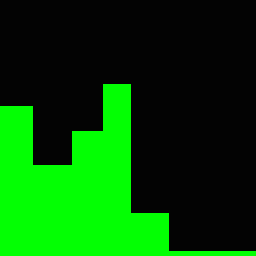Here is a histogram of a typical image’s green channel. We can by looking at it conclude that here are two major peaks, one at the black end and other at the middle tones. I’m using k-means clustering for the process. The general idea is to divide the distribution into separated masses, e.g. the columns in the image at right. The process is to first choose the clusters, in these case by dividing the color range of 0 to 255 to equal parts. The histogram values are each added to the nearest cluster. After that the new centroid of each cluster is updated. The process is repeated until it converges. In a one dimensional case like this, the sample space is only a integer range a 0 to 255 the update is simple: at current situation only the values at the edges are checked. The centroids of these columns should be a good estimate for the distribution of the histogram, since in this histogram there are less bright colors the last column is wider than the others.

Let’s remember that looking at the channels doesn’t of course solve the actual problem but gives a good approximation of how the color range is distributed in the RGB-space. Now that we know how the color ranges are distributed along each channel we can apply the same division to the entire rgb-space. Here’s images illustrating the process. The topmost one shows the color space distribution of an unmanipulated image, the middle one the result after the separation by channel, and the last is the result after the final clustering: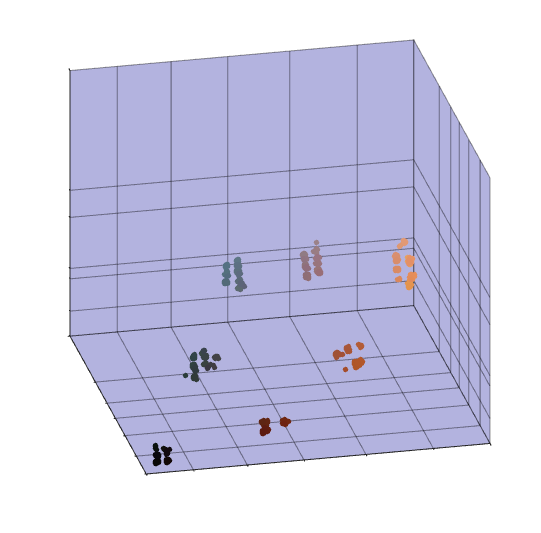Depending on the desired number of colors, the channels are divided to a maximum of eight clusters. Now stepping into the 3D, the maximum of bins is 8x8x8 = 512, and for the performance of a contemporary computer that should not be a challenge. Even as my implementation is very brute force, for every color bin it loops through all the centroids. However let’s remember, just as the images show, in a usual case of an input images, many areas in the rgb-space remain empty. In this demo I’m using javascript, for a flash implementation amount less than 256 would be an ideal solution: e.g. pack the entire color space to a single channel.

The final task is to map the colors of the input image into this new color space. In my code I’m using lookup tables, so the performance should be relatively fast.

Color Quantization has been a relatively popular subject of studies, and there’s plenty of solutions from different approaches … etc … but hey! About now it’s the time for the actual demo: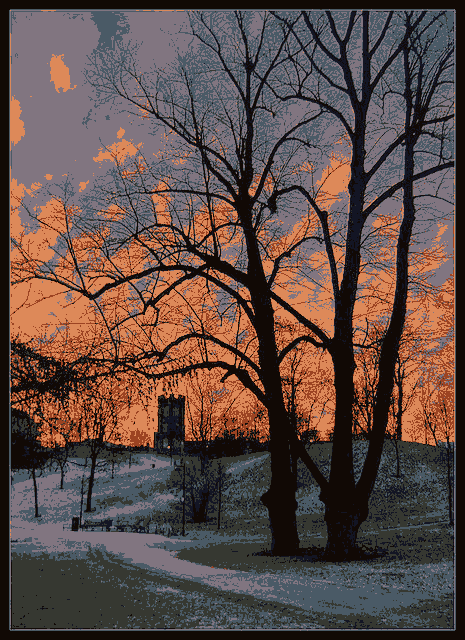You can test it for different levels of color, or upload an image of your own.

Javascript source code

Posted by: pixelero | November 18, 2011

## Laplacian Growth, vector graphicsGoing on with the same theme as in my last post, only that this time it’s all based on vector graphics.The principle is very loosely based on this article, or at least inspired by it …Demo

Posted by: pixelero | November 17, 2011

## Laplacian Growth, flash demo

Posted by: pixelero | November 17, 2011

## Pixel Bender: CubicSpace

Basicly it’s all about ray tracing on a rectangular grid: finding the intersection with the next cell wall:The projection used is like in panorama cameras, a cylinderic one. Try setting the parameter ‘focal length’ to a very small value and you’ll see things getting really ‘Escher’ or ‘Piranese’:

I admit it is a bit slow on performance …

Posted by: pixelero | July 5, 2011

## Pixel Bender: SplineAlignedImage – the Implicit Approach

In principle this is the same effect I already did with html5/canvas. Now it’s time for Pixel Bender to try some serious image bending.Click on the image above to go to the demo.

Ok, drawing a spline, or more generally rendering graphics along a spline, is relatively simple if you can explicitly calculate points of it and draw it part by part using lines, gradients or piecies of an image.
But it all becomes much more challening when in an environment, like Pixel Bender, where you can only go through the image pixel by pixel – and take the implicit approach.
If we where dealing with a line or a circle, calculating the distance to it would be so easy, but points on a Bezier are defined by a parametric cubic polynomial, and there’s no way to solve the inverse process algebrally. So what to do? There has to be some way to map the screen coordinates to a Bezier system: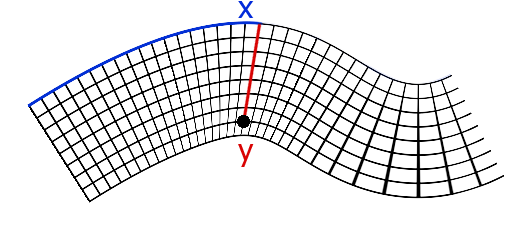Ok, looking at a picture always helps. Let’s say our current point is the black dot on picture and the Bezier passes on the top of the grid as the blue line.  First we find the nearest point on the curve, below ‘x’ where blue and red line meet. Distance to that, the red line, would be the new coordinate y. Then we calculate the distance along the path to that nearest point (blue line), length corresponds to coordinate x. Both of these calculation are ‘impossible’ to write as single evaluations, so we need some approximation.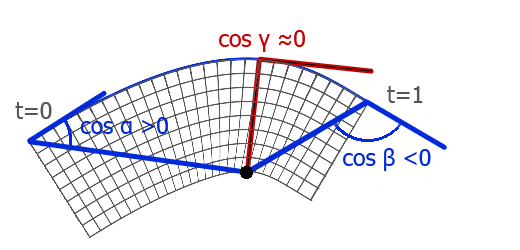From the curve we know the start and end points and the directions at both, so let’s start with this piece of information. We count, using dot product of two vectors, the cosine of angles between directions and the distance vectors to current point. If this value has different signs on the ends, current point lies somewhere in between, and we’re looking for the point where the value goes to zero.

Using values the start and end we approximate a point in between, repeating this a couple of times converges to a point where cos γ ≈ 0.0. For most cases this is a reliable result, although we totally neglected the fact that Beziers can even form closed loops, those cases would require splitting the curve to several sequences by points where x”=0 or y”=0. I mean the code should check out three candidates for the closest point.

My pixel bender-kernel repeates the iteration three times, which I think is adequate enough for the purpose of a computer graphics demonstration. In fact the method slightly differs from the principle I described, that’s only because also wanted to add the effect for rotating the image around spline’s main axis.

The mystery to solve here is a system of two nonlinear equations. You could also try solving that with like for instance Euler iteration using Jacobian determinate. The problem with that method is that it easily tends to converge to unwanted solutions, to points somewhere far from the actual spline sequence. Remember that Bezier is a continuous curve, where we only see the sequence from t=0 to t=1.

Ok, back to task. We now have the distance ‘y’. We need also the ‘x’, so all we need to do is just to calculate the distance from start point:But now wait a minute, that’s an Elliptic integral or something else as awful — unfortunately there’s no build-in function for it in Pixel Bender.  So we’ll use Simpson’s rule to get some idea of the length. In the simpliest case of that we calculate the sum of (value at start +3x (values at point of one third+value at point of two third) + value at end)/8. And to my surprise looking at the result it is enough!
I looked at the result with a more precise approach: sampled at 7 points with weights 1,3,3,2,3,3,1 – visually there was hardly any difference. In the demo same method is used when fitting an image’s width to spline’s length. It is said that Simpson perfectly approximates a cubic polynomial. In the formula x(t) and y(t) are cubic polynomials, which makes x'(t)² + y'(t)² of degree four, and square root of that could be something close to quadratic – at least that’s how I explained it to myself.As a conclusion. This is probably the stupidiest way of drawing by a spline: There are still errors in more ‘knotty’ cases. Furthermore the shader has to go through the entire bitmap to render even a smallest curve.

At least inside Flash Player much better results are obvious obtained by using methods of graphics like in my earlier post in case of 2D. Anyway I wanted to post it here, even if nothing else as an example of solving some awful mathematics.Posted by: pixelero | May 30, 2011

## Pixel Bender: Circular Disk Pattern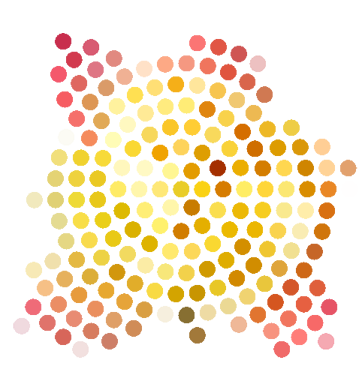Click on the images above to go to the demo.

After publishing some earlier posts like this I received an e-mail of how to create this kind of pattern.
After taking some time to consider the geometry and making a couple of attempts,
I came up with the code I’m now publishing.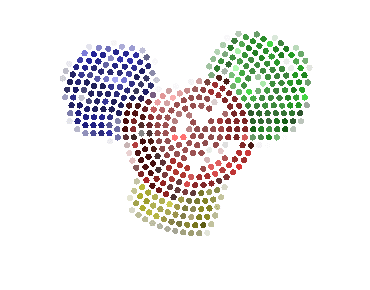View the sources here. Download sources.Pixel Bender -source code. Pixel Bender -filter.